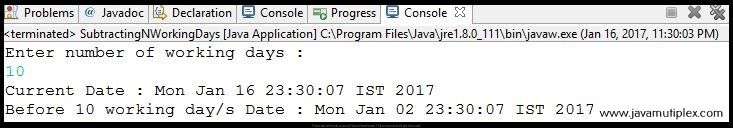## Problem Description :

Write a program in Java that subtracts N working days from the current date and prints new date with proper validations.

## Concept :

There are 5 working days per week (Monday, Tuesday, Wednesday, Thursday, Friday). Here in a loop we are subtracting 1 from the current date and checking day of that date. If it is Sunday or Saturday then we are doing increment to the value of N because we are interested in working days.
• We need to do subtraction from current date using add() method of Calendar class that accepts DAY_OF_MONTH constant and number of days that you want to subtract in negative format.
• For getting day, we need to use DAY_OF_WEEK constant of Calendar class.

## Recommended :

How to how to find every information about given date?

## Output :Output - How to subtract N working days from current date in Java?

## Similar Problem :

How to subtract N working days from given date in Java?

Solution - This is very simple first of all Convert given String to Date and rest is same as above.

## References :

### Thank you friends, I hope you have clearly understood the solution of this problem. If you have any doubt, suggestion or query please feel free to comment below. You can also discuss this solution in our forum. Tags : Date and Time problems, Subtraction of n working days in Java, Solution in Java, if else statement, for loop, Calendar.How to subtract N working days from the current date in Java?Reviewed by Rohit Agarwal on 1/16/2017 Rating: 5